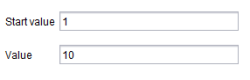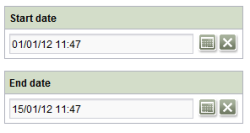# <ADD/>: Calculating with dynamic forms

The <ADD/> tag can be used in the value determination area of the rule definition (see <WITH/> tag) to:

• add integers to an existing value; for example: “2 + 3”
• add a time span, which is to be defined, to an existing date; for example: “01.01.2012 + 3 days”

## "Value" attribute

The “value” attribute is used to transfer an integer and (optionally) a time unit (separated by a space).

For this purpose, adding the following time units is supported:

• minute / minutes
• hour / hours
• day / days
• week / weeks
• month / months
• year / years

The integer and the time unit must be separated by a space; for instance:

`value=“2 weeks”`

## Examples

### Example 1) Adding an integerThe following form example shows two input windows labeled “st_startValue” and “st_value”. The purpose of the dynamic form is to add the value “9” automatically to an integer added by the editor in the “st_startValue” input component and to apply the new value in the “st_value” input component.

Validation does not take place in this example; a dependent input component is automatically populated with a value instead.

The value determination takes place within the <WITH/> tag. The <PROPERTY/> tag first takes the value from the “st_startValue” input component. The surrounding <ADD/> tags are used to add the value "9" to the existing value. Then the new value in the <DO/> section is applied to the “st_value” input component:

`<RULES>    <RULE>        <WITH>            <ADD value="9">                <PROPERTY source="st_startValue" name="VALUE"/>            </ADD>        </WITH>        <DO>            <PROPERTY source="st_value" name="VALUE"/>        </DO>    </RULE></RULES>`

### Example 2) Calculating a dateIn an additional form example, there is one entry field each for a start date (“st_startDate”) and an end date (“st_endDate”). Using a dynamic form, an end date is automatically calculated (+ 2 weeks) and applied to the “st_endDate” input component when the editor enters a start date.

Value determination takes place within the <WITH/> tag. The <PROPERTY/> tag first takes the value (date object) from the “st_startDate” input component. The surrounding <ADD/> tags are used to add the “2 weeks” time span to the existing date. Then the new date in the <DO/> section is applied to the “st_endDate” input component:

`<RULE>    <WITH>        <ADD value="2 weeks">            <PROPERTY source="st_startDate" name="VALUE"/>        </ADD>    </WITH>    <DO>        <PROPERTY source="st_endDate" name="VALUE"/>    </DO></RULE>`